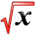# Riemann SumThis article is part of the MathHelp Tutoring Wiki

Riemann Sum is how integrals are calculated. It entails dividing the area under the curve being integrated into thin rectangles. When we want to integrate a function f(x) from x = a to x = b, ie, ∫_{a}^{b} f(x) dx, we want to divide the interval from a to b into N equal-width strips. Each strip has length (b-a)/N and height f(x). Riemann sum is the sum of the areas of these strips, and the resulting sum is equal to the result of the integration, if we let N --> .

For example, to calculate ∫_{-1}^{5} (1 + 3x) dx (we know the answer is x + 3x2/2 from -1 to 5, which is 42), we would divide the interval from -1 to 5 into N interval, so each interval has width 6/N. If we are to use the left-sided Riemann sum, the left side of the first rectangle is at x0 = -1, the second one at x1 = -1 + 6/N, third one at x2 = -1 + 2*6/N, fourth one at x3 = -1 + 3*(6/N), etc. The height of these rectangles are f(xi) = 1 + 3xi, ie, 1 + 3(-1), 1 + 3(-1+ 6/N), 1 + 3(-1 +2*6/N), 1 + 3(-1 + 3*6/N), respectively. In other words, for a general rectangle that starts at xi, the area of the rectangle is width (6/N) times height 1 + 3(-1 + 6i/N), or -12/N + 108i/N2. Now we sum up all the areas of the rectangles, ie, sigma_{i = 0}^{N-1} -12/N + 108i/N2.

The sum of the first term sigma_{i = 0}^{N-1} -12/N = -12 (because there are N terms of -12/N), and for the second term, rewrite it as 108/N2 * sigma_{i = 0}^{N-1} i = 108/N2 * N(N-1)/2 = 54N(N-1)/N2. Now take N --> ∞, the first term is -12, and the second term is 54. The sum is 42 as expected.

Example 1 :

4 rectangles (left hand end point)
Solution :
width = ${\frac {4}{4}}$= 1 * if 8 rectangles ${\frac {4}{8}}={\frac {1}{2}}$= width
Area = width x height
A = 1f(0) + 1f(1) + 1f(2) + 1f(3)
= 1[f(0) + f(1) + f(2) + f(3)]
= 1[0 + 1 + 4 + 9]
= 14

Example 2 :

4 rectangles (right hand end point)
Solution :
width = ${\frac {4}{4}}$= 1
Area = width x height
A = 1f(1) + 1f(2) + 1f(3) + 1f(4)
= 1[f(1) + f(2) + f(3) + f(4)]
= 1[1 + 4 + 9 + 16]
= 30

Example 3 :

A leak is found in the dam your company just finished constructing. The dam contains originally 10 million gallons of water and it is believed that the water is leaking out at a rate of r(t) = 0.23$e^{\frac {t}{1+t}}$millions of gallons per hour where t is the number of hours since the leak was found. Use Riemann sums to estimate the amount of water left in the dam.
Solution :
Use left end points with 5 subintervals.
Δt = ${\frac {b-a}{n}}$= ${\frac {5-0}{5}}$= 1
Riemann Sum = ((r(0) + r(1) + r(2) + r(3) + r(4))Δt
=(0.23$e^{\frac {0}{1+0}}$+ 0.23$e^{\frac {1}{2}}$+ 0.23$e^{\frac {2}{3}}$+ 0.23$e^{\frac {3}{4}}$+ 0.23$e^{\frac {4}{5}}$)(1)
=0.23($e^{0}$+ $e^{\frac {1}{2}}$+ $e^{\frac {2}{3}}$+ $e^{\frac {3}{4}}$+ $e^{\frac {4}{5}}$)
=2.0559 millions of gallons
The amount of water that leaks in the first 5 hours is approximately 2.0559 millions of gallons.
The amount of water left is 10 - 2.0559 = 7.9441